Next: Examples Up: Applications Previous: Envelope following   Contents   Index

Single Sideband Modulation

As we saw in Chapter 5, multiplying two real sinusoids together results in a signal with two new components at the sum and difference of the original frequencies. If we carry out the same operation with complex sinusoids, we get only one new resultant frequency; this is one result of the greater mathematical simplicity of complex sinusoids as compared to real ones. If we multiply a complex sinusoid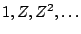with another one,the result is, which is another complex sinusoid whose frequency,, is the sum of the two original frequencies.

In general, since complex sinusoids have simpler properties than real ones, it is often useful to be able to convert from real sinusoids to complex ones. In other words, from the real sinusoid: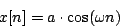(with a spectral peak of amplitudeand frequency) we would like a way of computing the complex sinusoid:so thatWe would like a linear process for doing this, so that superpositions of sinusoids get treated as if their components were dealt with separately.

Of course we could equally well have chosen the complex sinusoid with frequency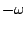: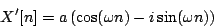and in fact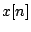is just half the sum of the two. In essence we need a filter that will pass through positive frequencies (actually frequencies between 0 and, corresponding to values ofon the top half of the complex unit circle) from negative values (fromto 0, or equivalently, fromto--the bottom half of the unit circle).

One can design such a filter by designing a low-pass filter with cutoff frequency, and then performing a rotation byradians using the technique of Section 8.3.4. However, it turns out to be easier to do it using two specially designed networks of all-pass filters with real coefficients.

Calling the transfer functions of the two filtersand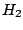, we design the filters so thator in other words,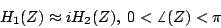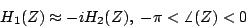Then for any incoming real-valued signalwe simply form a complex numberwhere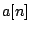is the output of the first filter and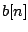is the output of the second. Any complex sinusoidal component of(call it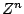) will be transformed toHaving started with a real-valued signal, whose energy is split equally into positive and negative frequencies, we end up with a complex-valued one with only positive frequencies.Next: Examples Up: Applications Previous: Envelope following   Contents   Index
Miller Puckette 2006-12-30